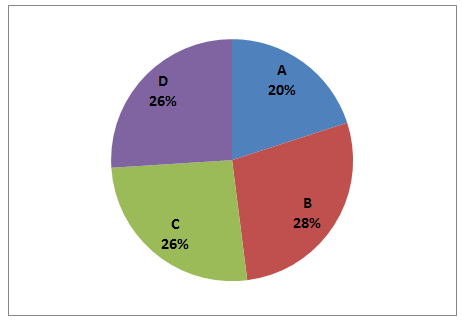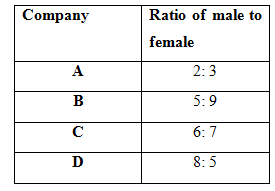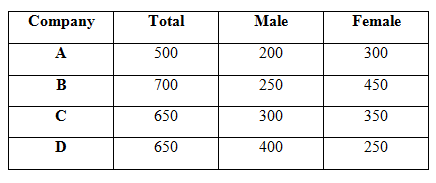# SBI Clerk Prelims 2021 Quantitative Aptitude Questions (Day-02)

Dear Aspirants, Our IBPS Guide team is providing new series of Quantitative Aptitude Questions for SBI Clerk Prelims 2020 so the aspirants can practice it on a daily basis. These questions are framed by our skilled experts after understanding your needs thoroughly. Aspirants can practice these new series questions daily to familiarize with the exact exam pattern and make your preparation effective.

Start Quiz

Directions (1-5): Find out the wrong number in the following number series.

1) 18, 20, 43, 133, 537, 2690

A.20

B.2690

C.43

D.537

E.133

2) 8, 20, 50, 122, 312.5, 781.25

A.20

B.50

C.122

D.312.5

E.None of these

3) 652, 636, 610, 575, 526, 462

A.575

B.636

C.462

D.610

E.526

4) 8, 14, 20, 50, 98, 194

A.20

B.50

C.98

D.194

E.None of these

5) 12, 88, 162, 234, 308, 372

A.308

B.372

C.88

D.234

E.162

Directions (6-10): Study the following information carefully and answer the questions given below.

The given below pie chart shows the percentage distribution of working employees in four different companies.

Total number of employees = 2500The given below table shows the ratio between the males and females distributed among four different companies.6) What is the total number of females working in the companies together?

A.1350

B.1450

C.1550

D.960

E.None of these

7) What is the difference between the number of males working in company B and the number of females working in company D?

A.0

B.20

C.40

D.60

E.None of these

8) Number of females working in company C is what percent more than the number of males working in company A?

A.25%

B.50%

C.75%

D.80%

E.None of these

9) What is the difference between the total number of employees working in company C and the number of females working in company B?

A.100

B.200

C.150

D.250

E.None of these

10) If 20% of males working in company C are married and the remaining males are unmarried, then what is the number of males working in company C who are unmarried?

A.120

B.240

C.280

D.180

E.None of these

Directions (1-5):

18 * 1 + 2 = 20

20 * 2 + 3 = 43

43 * 3 + 4 = 133

133 * 4 + 5 = 537

537 * 5 + 6 = 2691 (Not 2690)

8 * 2.5 = 20

20 * 2.5 = 50

50 * 2.5 = 125 (not 122)

125 * 2.5 = 312.5

312.5 * 2.5 = 781.25

652 – 42 = 636

636 – 52 = 611 (Not 610)

611 – 62 = 575

575 – 72 = 526

526 – 82 = 462

8 * 2 – 2 = 14

14 * 2 – 2 = 26 (not 20)

26 * 2 – 2 = 50

50 * 2 – 2 = 98

98 * 2 – 2 = 194

12 + 76 = 88

88 + 74 = 162

162 + 72 = 234

234 + 70 = 304 (Not 308)

304 + 68 = 372

Directions (6-10) :Required total = (300 + 450 + 350 + 250) = 1350

Required difference = 250 – 250

= 0

Required percentage = (350 – 200)/200 * 100

= 75%

Required difference = 650 – 450 = 200

Total employees in C = 650

Males working in C = 300

Married males in C = 20/100 * 300 = 60

Unmarried males in C = 300 – 60 = 240